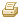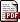Bellevue State Bank

# Rates

Time Certificates

Rates as of December 16, 2019

TERM

MINIMUM BALANCE

INTEREST RATE

ANNUAL PERCENTAGE YIELD

TERM: 30 Day

MINIMUM BALANCE: \$2,500

INTEREST RATE: 0.30%

ANNUAL PERCENTAGE YIELD: 0.30%

TERM: 91 Day

MINIMUM BALANCE: \$2,500

INTEREST RATE: 0.35%

ANNUAL PERCENTAGE YIELD: 0.35%

TERM: 182 Day

MINIMUM BALANCE: \$2,500

INTEREST RATE: 0.40%

ANNUAL PERCENTAGE YIELD: 0.40%

TERM: 6 Month

MINIMUM BALANCE: \$500

INTEREST RATE: 0.30%

ANNUAL PERCENTAGE YIELD: 0.30%

TERM: 10 Month

MINIMUM BALANCE: \$5,000

INTEREST RATE: 0.55%

ANNUAL PERCENTAGE YIELD: 0.55%

TERM: 11 Month

MINIMUM BALANCE: \$1,000

INTEREST RATE: 0.40%

ANNUAL PERCENTAGE YIELD: 0.40%

TERM: 1 Year

MINIMUM BALANCE: \$2,500

INTEREST RATE: 1.65%

ANNUAL PERCENTAGE YIELD: 1.66%

TERM: Less than \$2,500

MINIMUM BALANCE: \$500

INTEREST RATE: 0.45%

ANNUAL PERCENTAGE YIELD: 0.45%

TERM: 15 Month

MINIMUM BALANCE: \$2,500

INTEREST RATE: 0.90%

ANNUAL PERCENTAGE YIELD: 0.90%

TERM: 18 Month

MINIMUM BALANCE: \$5,000

INTEREST RATE: 1.00%

ANNUAL PERCENTAGE YIELD: 1.00%

TERM: 2 Year

MINIMUM BALANCE: \$2,500

INTEREST RATE: 1.70%

ANNUAL PERCENTAGE YIELD: 1.71%

TERM: Less than \$2,500

MINIMUM BALANCE: \$500

INTEREST RATE: 0.60%

ANNUAL PERCENTAGE YIELD: 0.60%

TERM: 30 Month

MINIMUM BALANCE: \$500

INTEREST RATE: 1.10%

ANNUAL PERCENTAGE YIELD: 1.10%

TERM: 3 Year

MINIMUM BALANCE: \$2,500

INTEREST RATE: 1.10%

ANNUAL PERCENTAGE YIELD: 1.10%

TERM: 4 Year

MINIMUM BALANCE: \$5,000

INTEREST RATE: 2.05%

ANNUAL PERCENTAGE YIELD: 2.06%

TERM: 5 Year

MINIMUM BALANCE: \$5,000

INTEREST RATE: 1.75%

ANNUAL PERCENTAGE YIELD: 1.76%

TERM: 35 Month - \$500 deposits

MINIMUM BALANCE: \$5,000

INTEREST RATE: 1.10%

ANNUAL PERCENTAGE YIELD: 1.10%

TERM: IRA** (APY may change January 1st and July 1st)

MINIMUM BALANCE: \$10

INTEREST RATE: 0.50%

ANNUAL PERCENTAGE YIELD: 0.50%

• Rates shown require a checking account
• A penalty may be charged for early withdrawal
• Minimum balance required to obtain the annual percentage yield

Checking and Savings Accounts* **

ACCOUNT

MINIMUM BALANCE

INTEREST RATE

ANUAL PERCENTAGE YIELD

ACCOUNT: Savings

MINIMUM BALANCE: \$100,000 and up

INTEREST RATE: 1.00%

ANNUAL PERCENTAGE YIELD: 1.00%

ACCOUNT:

MINIMUM BALANCE: \$10 to \$99,999

INTEREST RATE: 0.50%

ANNUAL PERCENTAGE YIELD: 0.50%

ACCOUNT: NOW

MINIMUM BALANCE: \$1,000

INTEREST RATE: 0.15%

ANNUAL PERCENTAGE YIELD: 0.15%

ACCOUNT: SUPER NOW

MINIMUM BALANCE: \$25,000 & Up

INTEREST RATE: 0.30%

ANNUAL PERCENTAGE YIELD: 0.30%

ACCOUNT: SUPER NOW

MINIMUM BALANCE: \$10,000 to \$24,999

INTEREST RATE: 0.20%

ANNUAL PERCENTAGE YIELD: 0.20%

ACCOUNT: SUPER NOW

MINIMUM BALANCE: \$2,500 to \$9,999

INTEREST RATE: 0.10%

ANNUAL PERCENTAGE YIELD: 0.10%

ACCOUNT: SUPER NOW

MINIMUM BALANCE: Less than \$2,500

INTEREST RATE: 0.05%

ANNUAL PERCENTAGE YIELD: 0.05%

ACCOUNT: MONEY MARKET

MINIMUM BALANCE: \$100,000 and up

INTEREST RATE: 1.00%

ANNUAL PERCENTAGE YIELD: 1.00%

ACCOUNT: MONEY MARKET

MINIMUM BALANCE: \$25,000 to \$99,999

INTEREST RATE: 0.75%

ANNUAL PERCENTAGE YIELD: 0.75%

ACCOUNT: MONEY MARKET

MINIMUM BALANCE: \$10,000 to \$24,999

INTEREST RATE: 0.35%

ANNUAL PERCENTAGE YIELD: 0.35%

ACCOUNT: MONEY MARKET

MINIMUM BALANCE: \$2,500 to \$9,999

INTEREST RATE: 0.20%

ANNUAL PERCENTAGE YIELD: 0.20%

ACCOUNT: MONEY MARKET

MINIMUM BALANCE: Less than \$2,500

INTEREST RATE: 0.10%

ANNUAL PERCENTAGE YIELD: 0.10%

ACCOUNT: PREFERRED PLUS

MINIMUM BALANCE: \$25,000 & up

INTEREST RATE: 0.30%

ANNUAL PERCENTAGE YIELD: 0.30%

ACCOUNT: PREFERRED PLUS

MINIMUM BALANCE: \$10,000 to \$24,999

INTEREST RATE: 0.20%

ANNUAL PERCENTAGE YIELD: 0.20%

ACCOUNT: PREFERRED PLUS

MINIMUM BALANCE: \$2,500 to \$9,999

INTEREST RATE: 0.10%

ANNUAL PERCENTAGE YIELD: 0.10%

ACCOUNT: PREFERRED PLUS

MINIMUM BALANCE: Less than \$2,500

INTEREST RATE: 0.05%

ANNUAL PERCENTAGE YIELD: 0.05%

Fees could reduce the earnings on your account.

*RATE INFORMATION: Your interest rate and annual percentage yield may change after the account is open.

**Minimum deposit of \$10.00 is required to open an account.

Minimum balance required to obtain the annual percentage yield.

We reserve the right to at any time require not less than 7 days notice in writing before any withdrawal from an interest bearing account other than a time deposit, or from any other savings account as defined by Regulation D.

PrintSave as PDF